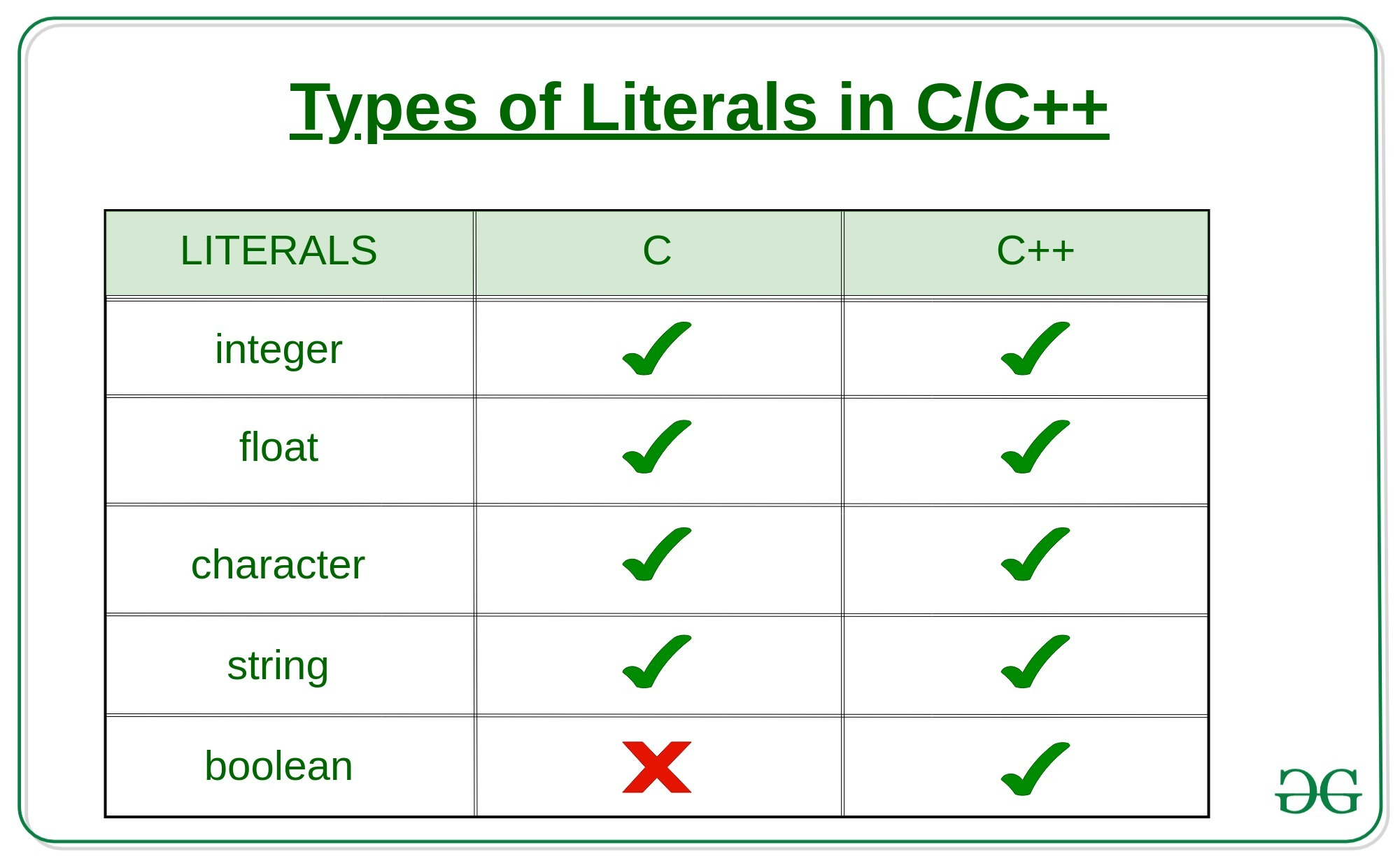Types of Literals in C/C++ with Examples

• Last Updated : 30 Sep, 2019

The values assigned to each constant variables are referred to as the literals. Generally, both terms, constants and literals are used interchangeably. For eg, “const int = 5;“, is a constant expression and the value 5 is referred to as constant integer literal. There are four types of literals in C and five types of literals in C++.The literals of C/C++ are divided accordingly:

1. Integer Literals: These are used to represent and store the integer values. Integer literals are expressed in two types i.e.,
1. Prefixes: The Prefix of the integer literal indicates the base in which it is to be read.
For example:
0x10 = 16

Because 0x prefix represents a HexaDecimal base.
So 10 in HexaDecimal is 16 in Decimal.
Hence the value 16.

There are basically represent in four types.

1. Decimal-literal(base 10): A non-zero decimal digit followed by zero or more decimal digits(0, 1, 2, 3, 4, 5, 6, 7, 8, 9).

For example:

56, 78

2. Octal-literal(base 8): a 0 followed by zero or more octal digits(0, 1, 2, 3, 4, 5, 6, 7).

For example:

045, 076, 06210

3. Hex-literal(base 16): 0x or 0X followed by one or more hexadecimal digits(0, 1, 2, 3, 4, 5, 6, 7, 8, 9, a, A, b, B, c, C, d, D, e, E, f, F).

For example:

0x23A, 0Xb4C, 0xFEA

4. Binary-literal(base 2): 0b or 0B followed by one or more binary digits(0, 1).

For example:

0b101, 0B111

2. Suffixes: The Prefix of the integer literal indicates the type in which it is to be read.

For example:

12345678901234LL
indicates a long long integer value 12345678901234
because of the suffix LL

These are represented in many ways according to their data types.

1. int: No suffix is required because integer constant is by default assigned as an int data type.
2. unsigned int: character u or U at the end of an integer constant.
3. long int: character l or L at the end of an integer constant.
4. unsigned long int: character ul or UL at the end of an integer constant.
5. long long int: character ll or LL at the end of an integer constant.
6. unsigned long long int: character ull or ULL at the end of integer constant.

Example:

C

 #include   int main(){      // constant integer literal    const int intVal = 10;      printf("Integer Literal:%d \n",           intVal);    return 0;}

C++

 #include using namespace std;  int main(){    // constant integer literal    const int intVal = 10;    cout << "Integer Literal: "         << intVal << "\n";      return 0;}
Output:

Integer Literal:10

2. Floating-Point Literals: These are used to represent and store real numbers. The real number has an integer part, real part, fractional part and an exponential part. The floating-point literals can be stored either in decimal form or exponential form. While representing the floating-point decimals one must keep two things in mind to produce valid literals:
• In the decimal form, one must include the decimal point, exponent part or both, otherwise, it will lead to an error.
• In the exponential form, one must include the integer part, fractional part or both, otherwise, it will lead to an error.

Few floating-point literal representations are shown below:

Valid Floating Literals:

10.125
1.215-10L
10.5E-3

Invalid Floating Literals:

123E
1250f
0.e879

Example:

C

 #include   int main(){    // constant float literal    const float floatVal = 4.14;      printf("Floating point literal: %.2f\n",           floatVal);    return 0;}

C++

 #include using namespace std;  int main(){    // Real literal    const float floatVal = 4.14;      cout << "Floating-point literal: "         << floatVal << "\n";    return 0;}
Output:
Floating point literal: 4.14

3. Character Literal: This refers to the literals that are used to store a single character within a single quote. To store multiple characters, one needs to use a character array. Storing more than one character within a single quote will throw a warning and displays just the last character of the literal. It gives rise to the following two representations:
• char type: This used to store normal character literal or the narrow-character literals. This is supported by both C and C++.

Example:

// For C
char chr = 'G';

// For C++
char chr = 'G';

• wchar_t type: This literal is suppoirted only in C++ and not in C. If the character is followed by L, then the literal needs to be stored in wchar_t. This represents wide-character literal.

Example:

// Not Supported For C

// For C++
wchar_t chr = L'G';

Example:

C

 #include   int main(){    // constant char literal    const char charVal = 'A';      printf("Character Literal: %c\n",           charVal);    return 0;}

C++

 #include using namespace std;  int main(){    // constant char literal    const char charVal = 'A';      // wide char literal    const wchar_t charVal1 = L'A';      cout << "Character Literal: "         << charVal << "\n";    cout << "Wide_Character Literal: "         << charVal1 << "\n";      return 0;}
Output:
Character Literal: A

Escape Sequences: There are various special characters that one can use to perform various operations.

4. String Literals: String literals are similar to that of the character literals, except that it can store multiple characters and uses a double quote to store the same. It can also accommodate the special characters and escape sequences mentioned in the table above.

Example:

// For C
char stringVal[] = "GeeksforGeeks";

// For C++
string stringVal = "GeeksforGeeks"

Example:

C

 #include   int main(){    const char str[]        = "Welcome\nTo\nGeeks\tFor\tGeeks";    printf("%s", str);    return 0;}

C++

 #include using namespace std;  int main(){    const string str        = "Welcome\nTo\nGeeks\tFor\tGeeks";    cout << str;    return 0;}
Output:
Welcome
To
Geeks    For    Geeks

5. Boolean Literals: This literal is provided only in C++ and not in C. They are used to represent the boolean datatypes. These can carry two values:
• true: To represent True value. This must not be considered equal to int 1.
• false: To represent False value. This must not be considered equal to int 0.

Example:

 // C++ program to show Boolean literals  #include using namespace std;  int main(){    const bool isTrue = true;    const bool isFalse = false;      cout << "isTrue? "         << isTrue << "\n";    cout << "isFalse? "         << isFalse << "\n";      return 0;}
Output:
isTrue? 1
isFalse? 0

Related articles on Constants/Literals in C/C++:

My Personal Notes arrow_drop_up